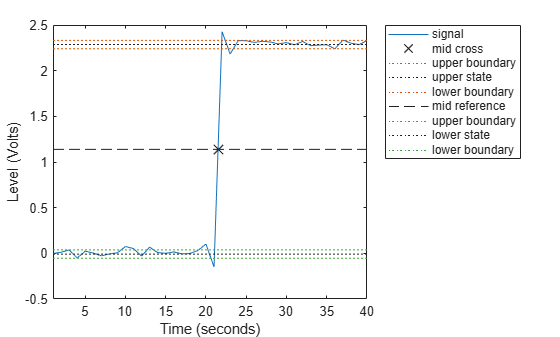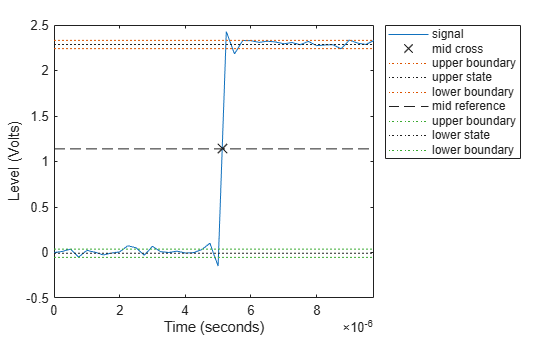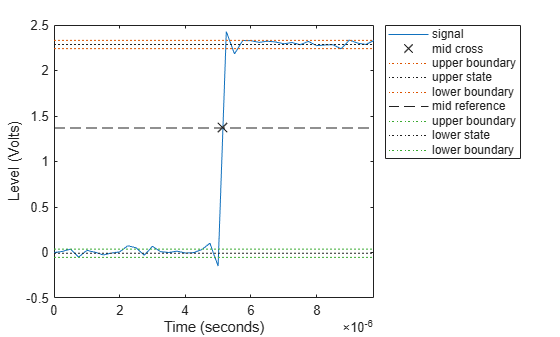Documentation

# midcross

Mid-reference level crossing for bilevel waveform

## Syntax

```C = midcross(X) C = midcross(X,FS) C = midcross(X,T) [C,MIDLEV] = midcross(...) C = midcross(X,Name,Value) midcross(...) ```

## Description

`C = midcross(X)` returns a vector, `C`, of time instants where each transition of the input signal, `X`, crosses the 50% reference level. The sample instants correspond to the indices of the input vector. Because `midcross` uses interpolation to determine the crossing instant, `C` may contain values that do not correspond to sampling instants. To determine the transitions, `midcross` estimates the state levels of `X` by a histogram method. `midcross` identifies all intervals which cross the upper-state boundary of the low state and the lower-state boundary of the high state. The low-state and high-state boundaries are expressed as the state level plus or minus a multiple of the difference between the state levels. See State-Level Tolerances.

`C = midcross(X,FS)` specifies the sample rate, `FS`, in hertz as a positive scalar. The first sample instant corresponds to t=0. Because `midcross` uses interpolation to determine the crossing instant, `C` may contain values that do not correspond to sampling instants.

`C = midcross(X,T)` specifies the sample instants, `T`, as a vector with the same number of elements as `X`. Because `midcross` uses interpolation to determine the crossing instant, `C` may contain values that do not correspond to sampling instants.

```[C,MIDLEV] = midcross(...)``` returns the waveform value corresponding to the mid-reference level.

`C = midcross(X,Name,Value)` returns the time instants corresponding to mid-reference level crossings with additional options specified by one or more `Name,Value` pair arguments.

`midcross(...)` plots the signal and marks the location of the mid-crossings (mid-reference level instants) and the associated reference levels. `midcross` also plots the state levels with upper and lower state boundaries.

## Input Arguments

 `X` Bilevel waveform. `X` is a real-valued row or column vector. `FS` Sample rate in hertz. `T` Vector of sample instants. The length of `T` must equal the length of the bilevel waveform, `X`.

### Name-Value Pair Arguments

Specify optional comma-separated pairs of `Name,Value` arguments. `Name` is the argument name and `Value` is the corresponding value. `Name` must appear inside quotes. You can specify several name and value pair arguments in any order as `Name1,Value1,...,NameN,ValueN`.

 `'MidPercentReferenceLevel'` Mid-reference level as a percentage of the waveform amplitude. Default: `50` `'StateLevels'` Low and high state levels. `StateLevels` is a 1-by-2 real-valued vector. The first element is the low state level. The second element is the high state level. If you do not specify low- and high-state levels, `midcross` estimates the state levels from the input waveform using the histogram method. `'Tolerance'` Tolerance levels (lower- and upper-state boundaries) expressed as a percentage. See State-Level Tolerances. Default: `2`

## Output Arguments

 `C` Time instants of the mid-reference level crossings. `MIDLEV` Mid-reference level.

## Examples

collapse all

Assuming a sampling interval of 1, compute the mid-reference level instant of a bilevel waveform. Plot the result.

```load('transitionex.mat','x') midcross(x)``````ans = 21.5000 ```

The instant at which the waveform crosses the 50% reference level is 21.5. This is not a sampling instant present in the input vector. `midcross` uses interpolation to identify the mid-reference level crossing.

Compute the mid-reference level instant for a sampled bilevel waveform. Use the time information to determine the sample rate, which is 4 MHz.

```load('transitionex.mat','x','t') Fs = 1/(t(2)-t(1))```
```Fs = 4000000 ```

Use the sample rate to express the mid-reference level instant in seconds. Plot the waveform and annotate the result.

`midcross(x,Fs)````ans = 5.1250e-06 ```

Compute the mid-reference level instant using a vector of sample times equal in length to the bilevel waveform. The sample rate is 4 MHz.

```load('transitionex.mat','x','t') C = midcross(x,t)```
```C = 5.1250e-06 ```

Annotate the result on a plot of the waveform.

`midcross(x,t);`Compute the level corresponding to the mid-reference level instant.

```load('transitionex.mat','x','t') [~,midlev] = midcross(x,t)```
```midlev = 1.1388 ```

Annotate the result on a plot of the waveform.

`midcross(x,t);`Obtain the 60% reference level instant and value for a bilevel waveform sampled at 4 MHz.

```load('transitionex.mat','x','t') [mc,Lev60] = midcross(x,t,'MidPercentReferenceLevel',60)```
```mc = 5.1473e-06 ```
```Lev60 = 1.3682 ```

Annotate the result on a plot of the waveform.

`midcross(x,t,'MidPercentReferenceLevel',60);`collapse all

### Mid-Reference Level

The mid-reference level in a bilevel waveform with low-state level, S1, and high–state level, S2, is

`${S}_{1}+\frac{1}{2}\left({S}_{2}-{S}_{1}\right)$`

### Mid Reference Level Instant

Let y50% denote the mid–reference level.

Let t50%- and t50%+ denote the two consecutive sampling instants corresponding to the waveform values nearest in value to y50%.

Let y50%- and y50%+ denote the waveform values at t50%- and t50%+.

The mid-reference level instant is

`${t}_{50%}={t}_{50%}+\left(\frac{{t}_{50{%}_{+}}-{t}_{50{%}_{-}}}{{y}_{50{%}_{+}}-{y}_{50{%}_{-}}}\right)\left({y}_{50{%}_{+}}-{y}_{50{%}_{-}}\right)$`

### State-Level Tolerances

Each state level can have associated lower- and upper-state boundaries. These state boundaries are defined as the state level plus or minus a scalar multiple of the difference between the high state and the low state. To provide a useful tolerance region, the scalar is typically a small number such as 2/100 or 3/100. In general, theregion for the low state is defined as

``

whereis the low-state level andis the high-state level. Replace the first term in the equation withto obtain thetolerance region for the high state.

The following figure illustrates lower and upper 2% state boundaries (tolerance regions) for a positive-polarity bilevel waveform. The red dashed lines indicate the estimated state levels.## References

 IEEE® Standard on Transitions, Pulses, and Related Waveforms, IEEE Standard 181, 2003. p. 20.